# Series Quiz III, Non Verbal Reasoning

## 25 Questions MCQ Test General Intelligence and Reasoning for SSC CGL | Series Quiz III, Non Verbal Reasoning

Description
Attempt Series Quiz III, Non Verbal Reasoning | 25 questions in 50 minutes | Mock test for LR preparation | Free important questions MCQ to study General Intelligence and Reasoning for SSC CGL for LR Exam | Download free PDF with solutions
QUESTION: 1

### Directions to Solve Each of the following questions consists of five figures marked A, B, C, D and E called the Problem Figures followed by five other figures marked 1, 2, 3, 4 and 5 called the Answer Figures. Select a figure from amongst the Answer Figures which will continue the same series as established by the five Problem Figures. Question - Select a figure from amongst the Answer Figures which will continue the same series as established by the five Problem Figures.Problem Figures:                          Answer Figures: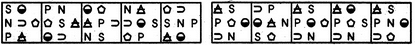(A)     (B)     (C)     (D)     (E)       (1)     (2)     (3)     (4)     (5)

Solution: In each step, all the symbols move in the sequence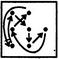QUESTION: 2

### Directions to Solve Each of the following questions consists of five figures marked A, B, C, D and E called the Problem Figures followed by five other figures marked 1, 2, 3, 4 and 5 called the Answer Figures. Select a figure from amongst the Answer Figures which will continue the same series as established by the five Problem Figures. Question - Select a figure from amongst the Answer Figures which will continue the same series as established by the five Problem Figures.Problem Figures:                          Answer Figures: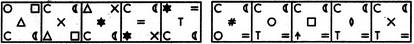(A)     (B)     (C)     (D)     (E)       (1)     (2)     (3)     (4)     (5)

Solution: The symbols move in the sequences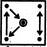and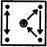alternately. In each step, the symbol that reaches the-encircled position gets replaced by a new symbol.
QUESTION: 3

### Directions to Solve Each of the following questions consists of five figures marked A, B, C, D and E called the Problem Figures followed by five other figures marked 1, 2, 3, 4 and 5 called the Answer Figures. Select a figure from amongst the Answer Figures which will continue the same series as established by the five Problem Figures. Question - Select a figure from amongst the Answer Figures which will continue the same series as established by the five Problem Figures.Problem Figures:                          Answer Figures: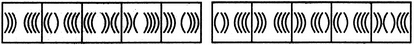(A)     (B)     (C)     (D)     (E)       (1)     (2)     (3)     (4)     (5)

Solution: Two, three, four, two, three,..... curves get inverted sequentially.
QUESTION: 4

Each of the following questions consists of five figures marked A, B, C, D and E called the Problem Figures followed by five other figures marked 1, 2, 3, 4 and 5 called the Answer Figures. Select a figure from amongst the Answer Figures which will continue the same series as established by the five Problem Figures.

Question -

Select a figure from amongst the Answer Figures which will continue the same series as established by the five Problem Figures.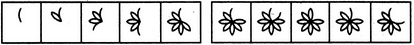(A)     (B)     (C)     (D)     (E)       (1)     (2)     (3)     (4)     (5)
Solution: Two and three half-leaves are added to the figure alternately. The addition of half-leaves takes place in an ACW direction.
QUESTION: 5

Each of the following questions consists of five figures marked A, B, C, D and E called the Problem Figures followed by five other figures marked 1, 2, 3, 4 and 5 called the Answer Figures. Select a figure from amongst the Answer Figures which will continue the same series as established by the five Problem Figures.

Question -

Select a figure from amongst the Answer Figures which will continue the same series as established by the five Problem Figures.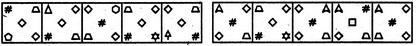(A)     (B)     (C)     (D)     (E)       (1)     (2)     (3)     (4)     (5)
Solution: The symbols move in the sequence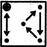in the first step. In each subsequent step, the symbols move in the sequence obtained by rotating the previous sequence through 90oCW. Also, in each step, the symbol that reaches the encircled position gets replaced by a new one.
QUESTION: 6

Each of the following questions consists of five figures marked A, B, C, D and E called the Problem Figures followed by five other figures marked 1, 2, 3, 4 and 5 called the Answer Figures. Select a figure from amongst the Answer Figures which will continue the same series as established by the five Problem Figures.

Question -

Select a figure from amongst the Answer Figures which will continue the same series as established by the five Problem Figures.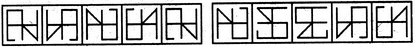(A)     (B)     (C)     (D)     (E)       (1)     (2)     (3)     (4)     (5)
Solution: The figure gets laterally inverted and vertically inverted alternately.
QUESTION: 7

Each of the following questions consists of five figures marked A, B, C, D and E called the Problem Figures followed by five other figures marked 1, 2, 3, 4 and 5 called the Answer Figures. Select a figure from amongst the Answer Figures which will continue the same series as established by the five Problem Figures.

Question -

Select a figure from amongst the Answer Figures which will continue the same series as established by the five Problem Figures.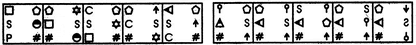(A)     (B)     (C)     (D)     (E)       (1)     (2)     (3)     (4)     (5)
Solution: The symbols move in the orders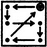and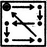alternately. Also, the symbol at the encircled position is replaced by a new one in each step.
QUESTION: 8

Each of the following questions consists of five figures marked A, B, C, D and E called the Problem Figures followed by five other figures marked 1, 2, 3, 4 and 5 called the Answer Figures. Select a figure from amongst the Answer Figures which will continue the same series as established by the five Problem Figures.

Question -

Select a figure from amongst the Answer Figures which will continue the same series as established by the five Problem Figures.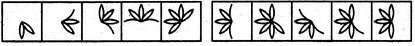(A)     (B)     (C)     (D)     (E)       (1)     (2)     (3)     (4)     (5)
Solution: In one step, a half-leaf is added to the figure at the ACW-end. In the next step, two half-leaves are added to the figure - one at the ACW-end and the other at the CW-end. Also, in each step, the figure rotates 45oACW.
QUESTION: 9

Each of the following questions consists of five figures marked A, B, C, D and E called the Problem Figures followed by five other figures marked 1, 2, 3, 4 and 5 called the Answer Figures. Select a figure from amongst the Answer Figures which will continue the same series as established by the five Problem Figures.

Question -

Select a figure from amongst the Answer Figures which will continue the same series as established by the five Problem Figures.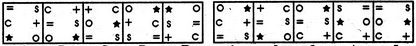(A)     (B)     (C)     (D)     (E)       (1)     (2)     (3)     (4)     (5)
Solution: The symbols '=', '+' and '*' move one step ACW While the symbols 's', 'c' and 'o' move one step CW each time.
QUESTION: 10

Each of the following questions consists of five figures marked A, B, C, D and E called the Problem Figures followed by five other figures marked 1, 2, 3, 4 and 5 called the Answer Figures. Select a figure from amongst the Answer Figures which will continue the same series as established by the five Problem Figures.

Question -

Select a figure from amongst the Answer Figures which will continue the same series as established by the five Problem Figures.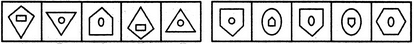(A)     (B)     (C)     (D)     (E)       (1)     (2)     (3)     (4)     (5)
Solution: Similar figure repeats in every third step and each time a figure reappears it gets vertically inverted.
QUESTION: 11

Directions to Solve Each of the following questions consists of five figures marked A, B, C, D and E called the Problem Figures followed by five other figures marked 1, 2, 3, 4 and 5 called the Answer Figures. Select a figure from amongst the Answer Figures which will continue the same series as established by the five Problem Figures.
Question - Select a figure from amongst the Answer Figures which will continue the same series as established by the five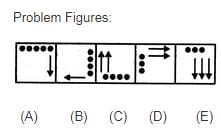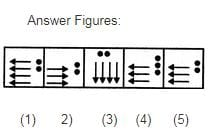Solution:

In each step, all the existing elements move to the adjacent side (of the square boundary) in a CW direction. The number of black circles decreases by one in first, third, fifth, .... steps and the number of arrows increases by one in second, fourth, sixth,... steps.

QUESTION: 12

Each of the following questions consists of five figures marked A, B, C, D and E called the Problem Figures followed by five other figures marked 1, 2, 3, 4 and 5 called the Answer Figures. Select a figure from amongst the Answer Figures which will continue the same series as established by the five Problem Figures.

Question -

Select a figure from amongst the Answer Figures which will continue the same series as established by the five Problem Figures.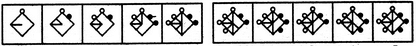(A)     (B)     (C)     (D)     (E)       (1)     (2)     (3)     (4)     (5)
Solution: In one step, a black circle is added to the figure at the CW-end of the existing circles and a line segment is added on the upper side. In the next step, a white circle is added to the figure at the ACW-end of the existing circles and a line segment is added on the lower side.
QUESTION: 13

Each of the following questions consists of five figures marked A, B, C, D and E called the Problem Figures followed by five other figures marked 1, 2, 3, 4 and 5 called the Answer Figures. Select a figure from amongst the Answer Figures which will continue the same series as established by the five Problem Figures.

Question -

Select a figure from amongst the Answer Figures which will continue the same series as established by the five Problem Figures.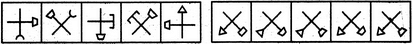(A)     (B)     (C)     (D)     (E)       (1)     (2)     (3)     (4)     (5)
Solution: The element having trapezium at its end, rotates 135oACW and the trapezium gets inverted in each step. The other element rotates 135oACW in one step and it rotates 45oCW and the symbol at its end gets replaced by a new element in the next step.
QUESTION: 14

Each of the following questions consists of five figures marked A, B, C, D and E called the Problem Figures followed by five other figures marked 1, 2, 3, 4 and 5 called the Answer Figures. Select a figure from amongst the Answer Figures which will continue the same series as established by the five Problem Figures.

Question -

Select a figure from amongst the Answer Figures which will continue the same series as established by the five Problem Figures.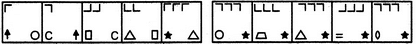(A)     (B)     (C)     (D)     (E)       (1)     (2)     (3)     (4)     (5)
Solution: The number of 'L' shaped elements in the upper part of the figure increases by one in every second step. Also, in each step, the existing 'L' shaped elements rotate 90oCW; the lower two elements interchange positions and the element that reaches the lower left corner, gets replaced by a new one.
QUESTION: 15

Each of the following questions consists of five figures marked A, B, C, D and E called the Problem Figures followed by five other figures marked 1, 2, 3, 4 and 5 called the Answer Figures. Select a figure from amongst the Answer Figures which will continue the same series as established by the five Problem Figures.

Question -

Select a figure from amongst the Answer Figures which will continue the same series as established by the five Problem Figures.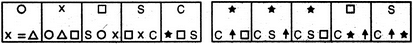(A)     (B)     (C)     (D)     (E)       (1)     (2)     (3)     (4)     (5)
Solution: The elements move in the sequences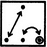and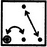alternately. Also, in each step, the element that reaches the encircled position, gets replaced by a new element.
QUESTION: 16

Each of the following questions consists of five figures marked A, B, C, D and E called the Problem Figures followed by five other figures marked 1, 2, 3, 4 and 5 called the Answer Figures. Select a figure from amongst the Answer Figures which will continue the same series as established by the five Problem Figures.

Question -

Select a figure from amongst the Answer Figures which will continue the same series as established by the five Problem Figures.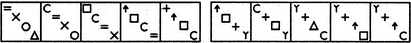(A)     (B)     (C)     (D)     (E)       (1)     (2)     (3)     (4)     (5)
Solution: In each step, the symbols move in the sequence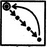and the symbol that reaches the encircled position gets replaced by a new symbol.
QUESTION: 17

Each of the following questions consists of five figures marked A, B, C, D and E called the Problem Figures followed by five other figures marked 1, 2, 3, 4 and 5 called the Answer Figures. Select a figure from amongst the Answer Figures which will continue the same series as established by the five Problem Figures.

Question -

Select a figure from amongst the Answer Figures which will continue the same series as established by the five Problem Figures.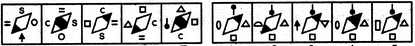(A)     (B)     (C)     (D)     (E)       (1)     (2)     (3)     (4)     (5)
Solution: The shading moves upwards sequentially from lower-left corner to the upper-right corner of the rhombus and once it reaches the upper-right corner, it moves to the lower-left corner in the next step. Also, in each step, all the symbols move to the adjacent side (of the rhombus) in a CW direction and the symbol that reaches the LHS position gets replaced by a new symbol.
QUESTION: 18

Each of the following questions consists of five figures marked A, B, C, D and E called the Problem Figures followed by five other figures marked 1, 2, 3, 4 and 5 called the Answer Figures. Select a figure from amongst the Answer Figures which will continue the same series as established by the five Problem Figures.

Question -

Select a figure from amongst the Answer Figures which will continue the same series as established by the five Problem Figures.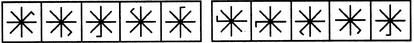(A)     (B)     (C)     (D)     (E)       (1)     (2)     (3)     (4)     (5)
Solution: The small line segment attached to the main figure moves 2,1, 3,1, 4,... spaces CW sequentially.
QUESTION: 19

Each of the following questions consists of five figures marked A, B, C, D and E called the Problem Figures followed by five other figures marked 1, 2, 3, 4 and 5 called the Answer Figures. Select a figure from amongst the Answer Figures which will continue the same series as established by the five Problem Figures.

Question -

Select a figure from amongst the Answer Figures which will continue the same series as established by the five Problem Figures.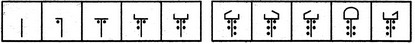(A)     (B)     (C)     (D)     (E)       (1)     (2)     (3)     (4)     (5)
Solution: One dot and one line segment are added to the figure in each step, once to the LHS and next to the RHS part of the figure.
QUESTION: 20

Each of the following questions consists of five figures marked A, B, C, D and E called the Problem Figures followed by five other figures marked 1, 2, 3, 4 and 5 called the Answer Figures. Select a figure from amongst the Answer Figures which will continue the same series as established by the five Problem Figures.

Question -

Select a figure from amongst the Answer Figures which will continue the same series as established by the five Problem Figures.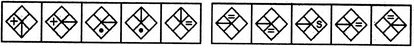(A)     (B)     (C)     (D)     (E)       (1)     (2)     (3)     (4)     (5)
Solution: The line segment rotates through 90o and moves to the adjacent portion of the rhombus in an ACW direction in first, third, fifth, ... steps. The other symbol moves to the adjacent portion of the rhombus in an ACW direction and also gets replaced by a new symbol in second, fourth, sixth,... steps.
QUESTION: 21

Each of the following questions consists of five figures marked A, B, C, D and E called the Problem Figures followed by five other figures marked 1, 2, 3, 4 and 5 called the Answer Figures. Select a figure from amongst the Answer Figures which will continue the same series as established by the five Problem Figures.

Question -

Select a figure from amongst the Answer Figures which will continue the same series as established by the five Problem Figures.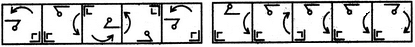(A)     (B)     (C)     (D)     (E)       (1)     (2)     (3)     (4)     (5)
Solution: The bent pin gets laterally inverted and vertically inverted alternately and moves to the adjacent side (of the square boundary) in a CW direction in each step,. The curved arrow rotates 90oACW and moves to the adjacent side CW in each step. As for the third element - in one step the outer 'L' shaped symbol gets laterally inverted and in the next step the element gets vertically inverted. Or, similar figure reappears in every fourth step. So, after fig. (E), the fig. (B) should reappear.
QUESTION: 22

Each of the following questions consists of five figures marked A, B, C, D and E called the Problem Figures followed by five other figures marked 1, 2, 3, 4 and 5 called the Answer Figures. Select a figure from amongst the Answer Figures which will continue the same series as established by the five Problem Figures.

Question -

Select a figure from amongst the Answer Figures which will continue the same series as established by the five Problem Figures.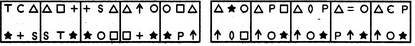(A)     (B)     (C)     (D)     (E)       (1)     (2)     (3)     (4)     (5)
Solution: The elements move in the sequences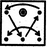and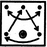alternately. Also, in each step, the element at the encircled position gets replaced by a new element.
QUESTION: 23

Each of the following questions consists of five figures marked A, B, C, D and E called the Problem Figures followed by five other figures marked 1, 2, 3, 4 and 5 called the Answer Figures. Select a figure from amongst the Answer Figures which will continue the same series as established by the five Problem Figures.

Question -

Select a figure from amongst the Answer Figures which will continue the same series as established by the five Problem Figures.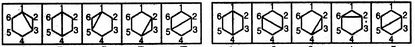(A)     (B)     (C)     (D)     (E)       (1)     (2)     (3)     (4)     (5)
Solution: The diagonals of the hexagon are drawn in a sequence - (1, 3), (1, 4), (1, 5), (2, 4), (2, 5), (2, 6), (3, 5), (3, 6), (4, 6).
QUESTION: 24

Each of the following questions consists of five figures marked A, B, C, D and E called the Problem Figures followed by five other figures marked 1, 2, 3, 4 and 5 called the Answer Figures. Select a figure from amongst the Answer Figures which will continue the same series as established by the five Problem Figures.

Question -

Select a figure from amongst the Answer Figures which will continue the same series as established by the five Problem Figures.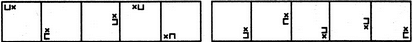(A)     (B)     (C)     (D)     (E)       (1)     (2)     (3)     (4)     (5)
Solution: The two elements together move two spaces (each space is equal to half-a-side of the square boundary) and three space ACW alternately. Also, in one step, the two elements interchange positions and together rotate 90oCW and in the next step, the two elements interchange positions and together rotate through 180o.
QUESTION: 25

Each of the following questions consists of five figures marked A, B, C, D and E called the Problem Figures followed by five other figures marked 1, 2, 3, 4 and 5 called the Answer Figures. Select a figure from amongst the Answer Figures which will continue the same series as established by the five Problem Figures.

Question -

Select a figure from amongst the Answer Figures which will continue the same series as established by the five Problem Figures.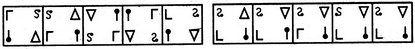Use Code STAYHOME200 and get INR 200 additional OFF Use Coupon Code